# SAT II Math II : Parabolas

## Example Questions

### Example Question #1 : Coordinate Geometry

Which of the following equations represent a parabola?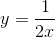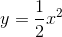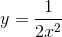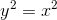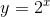The parabola is represented in the form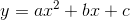.  If there is a variable in the denominator or as an exponent, it is not a parabola.
The only equation that has an order of two is: